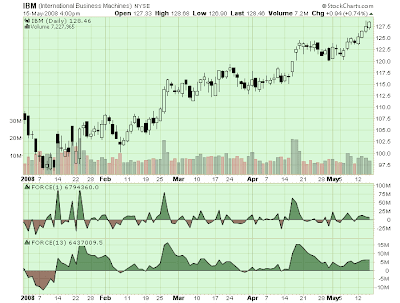# Force Index

Developed by Alexander Elder, the Force Index is a price-and-volume oscillator that helps technical analysts determine if a stock's trend is strengthening or weakening.

## Calculation

The raw Force Index is calculated as the difference between today's close and yesterday's close times today's volume. The "FORCE(1)" indicator in the chart below is an example of the raw Force Index:Because the raw Force Index is so choppy, many people use an EMA to smooth it. The 13-period EMA is the most popular choice for smoothing the Force Index. You can see the difference between the raw Force Index and the smoothed Force Index above.

## Interpretation

Like all oscillators, the Force Index generates buy and sell signals when it crosses it's center line. Trendlines can also be used to determine the direction and strength of the indicator's current movement. When the Force Index is setting new highs, the stock's current uptrend is likely to continue. When it is setting new low's, the stock will probably keep sinking. If the Force Index is moving sideways, a trend change is likely.

## Parameters

In SharpCharts, the Force Index can be found in the "Indicators" dropdowns. It takes one parameter which is the number of periods to use when exponentially smoothing the raw Force Index. The default smoothing value is 13. You can see the raw Force Index by using "1" as the parameter.